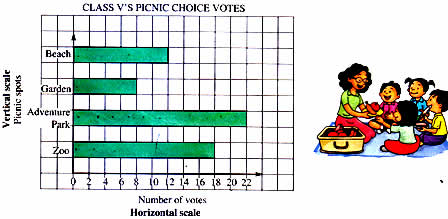Tuesday , July 5 2022# NCERT 5th Class (CBSE) Mathematics: Graphical Representation

## BAR GRAPHS

Another way to represent data is to show it in the form of bar graphs.

1. Bar graphs represent numerical information with the help of columns or bars.
2. It has a title explaining the information given in the graph.
3. It has horizontal and vertical scales with labels explaining the scales.

(a) Class V children voted on where they would like to go for their class picnic.

Beach – 12 voted

This data can be shown as a horizontal bar graph. Here each square represents two votes.In a bar graph, it is easier to first read what is on the vertical scale – for example, ‘beach’. Then from the point where the bar related to related to beach ends, go downwards to the horizontal scale to read the number –

12 student. This tells us that 12 students voted for beach as their choice for a picnic.

(b) Given below are the marks that Srinivas got in four maths tests. The testes were of 50 marks.

## चुनौती हिमालय की 5th NCERT CBSE Hindi Rimjhim Ch 18

चुनौती हिमालय की 5th Class NCERT CBSE Hindi Book Rimjhim Chapter 18 प्रश्न: लद्दाख जम्मू-कश्मीर राज्य में …